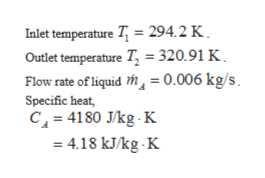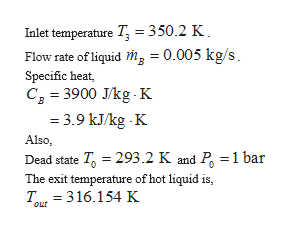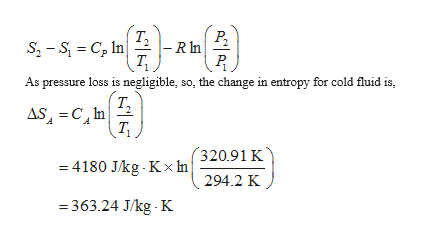# A heat exchanger is being investigated as a waste heat recovery device. A heat exchanger is common device for using a hot fluid to heat a cold fluid without the fluids mixing.  The following information is known.  The cold fluid stream of liquid A enters at 294.2 K and leaves the device at a temperature of 320.91 K.  Liquid A flows at a rate of 0.006 Kg/s and has a specific heat of 4180 J/(Kg K).  Liquid B enters the device at a temperature of 350.2 K.  Liquid B flows at a rate of 0.005 Kg/s and has a specific heat of 3900 J/(Kg K  The dead state is at 293.2 K and 1 bar.  The device is adiabatic and the pressure losses are neglected in this problem.  Both liquids are incompressible materials. Tout=316.154KAnswer the following: If the exiting liquid B is not used for any useful purpose, calculate the exergy destroyed associated with it.

Question
52 views

A heat exchanger is being investigated as a waste heat recovery device. A heat exchanger is common device for using a hot fluid to heat a cold fluid without the fluids mixing.  The following information is known.  The cold fluid stream of liquid A enters at 294.2 K and leaves the device at a temperature of 320.91 K.  Liquid A flows at a rate of 0.006 Kg/s and has a specific heat of 4180 J/(Kg K).  Liquid B enters the device at a temperature of 350.2 K.  Liquid B flows at a rate of 0.005 Kg/s and has a specific heat of 3900 J/(Kg K  The dead state is at 293.2 K and 1 bar.  The device is adiabatic and the pressure losses are neglected in this problem.  Both liquids are incompressible materials. Tout=316.154K

If the exiting liquid B is not used for any useful purpose, calculate the exergy destroyed associated with it.

check_circle

Step 1

Consider the case for cold fluid.

Here,help_outlineImage TranscriptioncloseInlet temperature T 294.2 K Outlet temperature T = 320.91 K Flow rate of liquid h, = 0.006 kg/s Specific heat, C 4180 J/kg K 4.18 kJkg. K fullscreen
Step 2

Consider the case for hot fluid.

Here,help_outlineImage TranscriptioncloseInlet temperature T =350.2 K Flow rate of liquid mg = 0.005 kg/s Specific heat, C 3900 J/kg K 3.9 kJ/kg K Also Dead state T 293.2 K and P 1 bar The exit temperature of hot liquid is T 316.154 K Out fullscreen
Step 3

The change in en...help_outlineImage Transcriptioncloseт, - R n Р. S2-S C In T As pressure loss is negligible, so, the change in entropy for cold fluid is, Т, AS C In 320.91 K 4180 J/kg Kx In 294.2 K =363.24 J/kg K fullscreen

### Want to see the full answer?

See Solution

#### Want to see this answer and more?

Solutions are written by subject experts who are available 24/7. Questions are typically answered within 1 hour.*

See Solution
*Response times may vary by subject and question.
Tagged in

### Heat Transfer